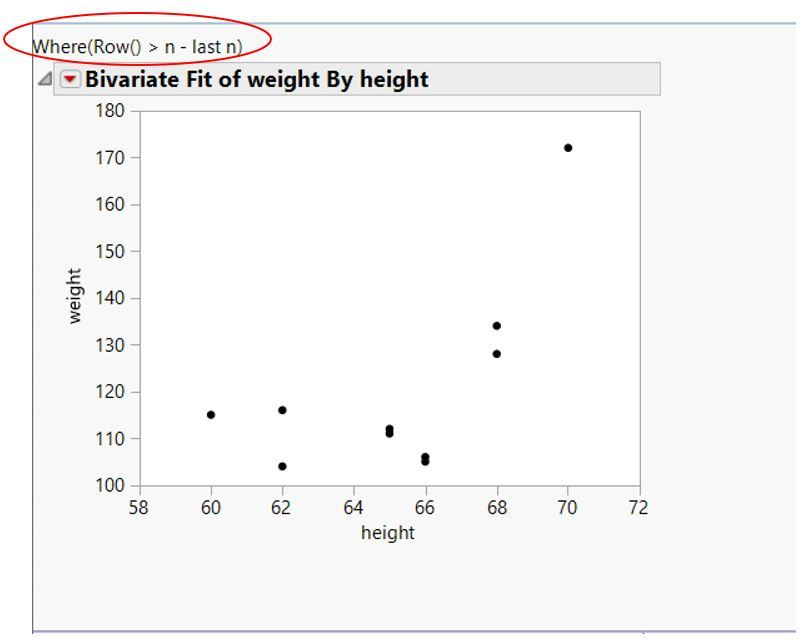Our World Statistics Day conversations have been a great reminder of how much statistics can inform our lives. Do you have an example of how statistics has made a difference in your life? Share your story with the Community!
Choose Language Hide Translation Bar
Highlighted

## JSL to plot graph with last N values of a column

I am writing script to plot graph with last 10 values of a column. Below is the script I use which I learned from previous post.

It works. But I notice the this wording " Where( Row() > (n - last n) ) " appear in the graph which I do not want it to show.

Is there a way to remove this wording? or Is there other way to write JSL script to plot graph with last N values of a column?

``````dt = Open( "\$SAMPLE_DATA/Big Class.jmp" );

last n = 10;
n = N Row( dt );

biv = dt << Bivariate(
Y( :weight ),
X( :height ),
Where( Row() > (n - last n) )
);``````1 ACCEPTED SOLUTION

Accepted Solutions
Highlighted

## Re: JSL to plot graph with last N values of a column

Here is a quick example.  It was just recently covered in a Discussion Here

``````dt = Open( "\$SAMPLE_DATA/Big Class.jmp" );

last n = 10;
n = N Row( dt );

biv = dt << Bivariate(
Y( :weight ),
X( :height ),
Where( Row() > (n - last n) )
);
(report(biv)<<topparent)[textbox(1)]<<delete;``````
Jim
2 REPLIES 2
Highlighted

## Re: JSL to plot graph with last N values of a column

Here is a quick example.  It was just recently covered in a Discussion Here

``````dt = Open( "\$SAMPLE_DATA/Big Class.jmp" );

last n = 10;
n = N Row( dt );

biv = dt << Bivariate(
Y( :weight ),
X( :height ),
Where( Row() > (n - last n) )
);
(report(biv)<<topparent)[textbox(1)]<<delete;``````
Jim
Highlighted

## Re: JSL to plot graph with last N values of a column

works great. Thanks

Article Labels